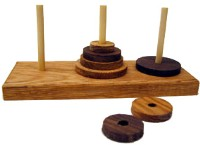Mathwire.com
Problem Solving Resources

## Kindergarten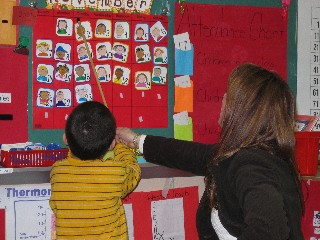These problems are designed for students in kindergarten.

•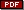Pattern Problems: visual patterns that require students to draw what comes next.   Teachers should ask students to explain how they know what comes next to develop students' ability to explain their thinking.
•Animal Problems ask students to solve problems about pets and animals.
•Shape Patterns: shape patterns that require students to draw what comes next.   Teachers should ask students to explain how they know what comes next to develop students' ability to explain their thinking.
•Food Problems: students solve problems that relate to food, drawing to figure out how many cookies, pizza, cupcakes were eaten in all.
•Winter Fun problems use snowmen, sleds and mittens to engage kindergarteners in problem solving.
•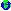Math Practice Problems for Kindergarten
•Math Worksheets for K-8 students

## Grades 1-2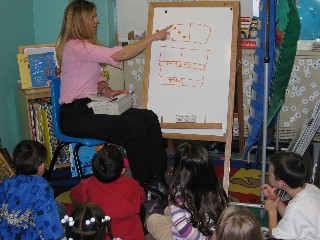These problems are designed for students in Grades 1-2:

•Largest Sum challenges students to arrange given digits to produce the largest sum.
•Smallest Sum challenges students to arrange given digits to produce the smallest sum.
•Largest Differencechallenges students to arrange the given digits to produce the largest difference.
•Smallest Difference challenges students to arrange the given digits to produce the smallest difference.
•Bean Bags assesses student understanding of place value using base-ten longs and cubes.
•Birthdays requires students to interpret data in a bar graph.
•Class Gardens measures student understanding of area and perimeter.
•Fair Game assesses student understanding of probability through in spinner games.
•Odd-Even Game requires students to interpret data in a tally chart.
•Pet Survey requires that students use data in a frequency table to construct a bar graph and answer questions about the graph.
•Math Practice Problems for Grade 1
•Math Practice Problems for Grade 2
•Math Stars Newsletters: problem solving for talented math students
•Math Worksheets for K-8 students

## Grades 3-4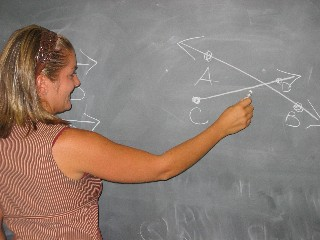These problems are designed for students in Grades 3-4:

•Pattern Block Fraction Design requires students to fill a shape with pattern blocks to create a design that meets certain requirements.   Students must also write a fraction that describes the part of the total design represented by each different color pattern block.
•Spinner Game presents students with a frequency table of spins and requires students to create a bar graph of the results and draw a spinner that would yield those results.   Students must justify their proposed spinner, explaining how it fits the data.
•Fraction Game simulates a Fraction War game but students must draw a representation of each fraction and explain who won, based on the drawings.
•Pattern Block Symmetry challenges students to use pattern blocks to fill in the shape so that the design has a vertical or horizontal line of symmetry.
•Pattern Block Symmetry: students must create a pattern block design to satisy the given conditions involving line symmetry and the number of different pattern block pieces used.
•Pattern Block Design (Grade 3) requires students to create a design to meet specified criteria.
•Pattern Block Design (Grade 4) requires students to create a design to meet specified criteria.
•Coordinate Geometry (Grade 4) requires students to plot specified points, connect the points to form a figure, then answer geometric questions about the figure.
•Field Trip (Grade 4) requires students to solve a real-life division problem and decide how to handle the remainder.
•Ants Marching assesses student understanding of the concept of multiplication as arrays.
•Third Grade Parade also assesses student understanding of the concept of multiplication as arrays.
•Garage Sale is a pattern problem based on dominoes.
•TV Survey is an example of a data analysis problem with questions designed to address each level of Bloom's Taxonomy.
•Largest 3-digit Sum challenges students to arrange given digits to form the largest sum and explain their reasoning.
•Smallest 3-digit Difference challenges students to arrange given digits to form the smallest difference and explain their reasoning.
•High-Number Toss - 1 is designed to measure student understanding of place value as it is used in the Everyday Math Game of the same name.   Students must be familiar with the game to successfully solve the problem.
•High-Number Toss - 2 is designed to measure student understanding of place value as it is used in the Everyday Math Game of the same name.   Students must be familiar with the game to successfully solve the problem.
•Name That Number - 1 is designed to measure student understanding of place value as it is used in the Everyday Math Game of the same name.   Students must be familiar with the game to successfully solve the problem.
•Name That Number - 2 is designed to measure student understanding of place value as it is used in the Everyday Math Game of the same name.   Students must be familiar with the game to successfully solve the problem.
•Favorite Shells is a pattern problem that can be solved using a picture or an input/output table.
•Area and Perimeter - 1 was designed to assess student understanding of the difference between area and perimeter.
•Area and Perimeter - 2 was designed to assess student understanding of the difference between area and perimeter.
•Shape Investigations was designed to help students develop the concept that two figures that have the same perimeter do not necessarily have the same area and vice versa.
•Fall Parade
•Marathon Training is a pattern problem that encourages students to use an input/output table to organize the data and the solution.
•Pumpkin Picking is another pattern problem that can be solved using a picture or an input/output table. contributed by Shannon Collier, Joseph C. Caruso School, Keansburg, NJ
•Baseball Season is a pattern problem that can be easily solved using a table of values.
•Anthony's Allowance provides additional pattern practice.
•Cheerleader Competition was designed to assess student understanding of multiplication as an array.
•Open-ended Math Problems from the Franklin Institute Online offers monthly problems in Number Theory; Geometry; Measurement; Patterns, Algebra & Functions; Data, Statistics & Probability.   The site offers three different levels of difficulty for each strand.
•Math Practice Problems for Grade 3
•Grade 3 Math Inventory: Web School
•Math Practice Problems for Grade 4
•Math Stars Newsletters: problem solving for talented math students
•Math Worksheets for K-8 students

## Grades 5-6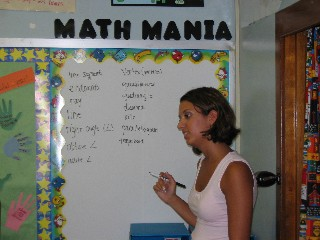These problems are designed for students in Grades 5-6.

•Bake Sale requires students to work backward to solve the problem.
•Monkey Business also requires students to work backward to figure out how many coconuts there were before each monkey ate one and took a third of what was left.
•Reading Challenge requires students to figure out what page a student read last, given only the product of the last two pages.
•Factor Investigation challenges students to list all factors of the numbers 1-25 and identify the numbers as abundant, deficient, perfect, prime.   Students can refer to this list when playing Factor Blaster or Factor Game.
•Thirteen Ways Recording Sheet was designed for students to record the thirteen ways to shade the diagram to represent 1/2 as found on the PBS Cyberchase website listed on the Recording sheet.
•MATH TV: Problem Solving Videos are designed for middle school students.   Students may choose from several interactive word problems and then view the video solution for each.
•Open-ended Math Problems from the Franklin Institute Online offers monthly problems in Number Theory; Geometry; Measurement; Patterns, Algebra & Functions; Data, Statistics & Probability.   The site offers three different levels of difficulty for each strand.
•Math Practice Problems for Grade 5
•Grade 5 Math Inventory: Web School
•Math Practice Problems for Grade 6
•Puzzling and Perplexing Problems:   Seasonal Math Problems for the Middle School Math Class
•The Million Dollar Mission asks students to decide which salary is the better offer for one month's work: one million dollars or one cent on the first day, two cents on the second day, four cents on the third day, etc.
•Math Stars Newsletters: problem solving for talented math students
•Math Worksheets for K-8 students

## Grades 7-8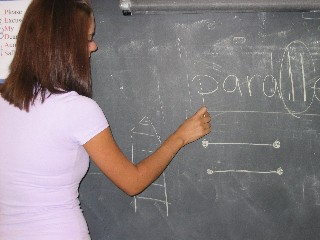These problems were designed for students in Grades 7-8.

•Investigating Exponents challenges students to identify the different patterns found in the units digits of numbers raised to different powers.
•MATH TV: Problem Solving Videos are designed for middle school students.   Students may choose from several interactive word problems and then view the video solution for each.
•Open-ended Math Problems from the Franklin Institute Online offers monthly problems in Number Theory; Geometry; Measurement; Patterns, Algebra & Functions; Data, Statistics & Probability.   The site offers three different levels of difficulty for each strand.
•Math Practice Problems for Grade 7
•Math Practice Problems for Grade 8
•New York Sample 8th Grade Open Response Test
•New York Sample 8th Grade Open Response Test Solutions
•Grade 8 Math Inventory: Web School
•Puzzling and Perplexing Problems:   Seasonal Math Problems for the Middle School Math Class
•The Million Dollar Mission asks students to decide which salary is the better offer for one month's work: one million dollars or one cent on the first day, two cents on the second day, four cents on the third day, etc.
•Math Stars Newsletters: problem solving for talented math students
•Math Worksheets for K-8 students

## Rubrics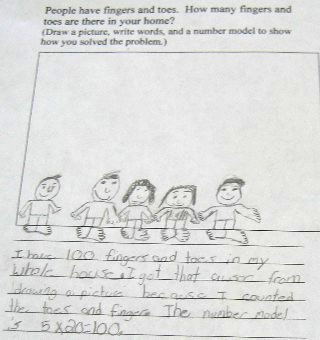These links are general problem-solving rubrics in different formats.## Links to Problem Solving sites

These links provide additional problem-solving activities for students in all grades.

These links challenge students to develop logical thinking and use patterns to solve these puzzles and brain teasers.